Courses

# Variational Principle : Euler-Lagrange Equation and its Applications (Part -2) - Classical Mechanics Physics Notes | EduRev

## Physics for IIT JAM, UGC - NET, CSIR NET

Created by: Akhilesh Thakur

## Physics : Variational Principle : Euler-Lagrange Equation and its Applications (Part -2) - Classical Mechanics Physics Notes | EduRev

The document Variational Principle : Euler-Lagrange Equation and its Applications (Part -2) - Classical Mechanics Physics Notes | EduRev is a part of the Physics Course Physics for IIT JAM, UGC - NET, CSIR NET.
All you need of Physics at this link: Physics

Example: Planetary Motion

Consider the motion of a planet due to the force exerted by Sun. Using polar coordinates we can write the Lagrangian for the planet as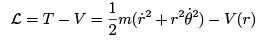We have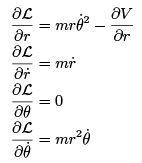The Euler- Lagrange equation for the angle variable is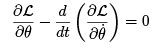which gives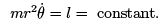(8)

This is the statement of conservation of energy which follows from Kepler's second law.
The equation for the radial variable is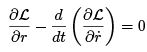from which we get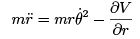Substituting from (8), we can write this as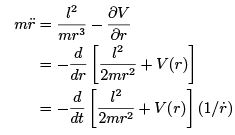From the above we get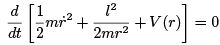(9)

which is a statement of conservation of energy.

Validity of Euler Lagrange Equation in Generalized Coordinates

t was seen that the degrees of freedom of two particles connected by a rigid rod is 5, and not 6, as the constraint of keeping the length xed bewteen the two masses reduces the number of independent quantities by 1. It is easy to see that a rigid body has 6 degrees of freedom, independent of the number of particles N . This is seen by the following argument. Starting with two particles, which has ve degrees of freedom, add a third particle non-collinear with the former two. This should increase the degree of freedom by 3. However, we have now introduced two additional constraints, viz., the distance between this newly added particle and the former two must remain constant. These two constraints, therefore, add only one additional degree of freedom and not three. Thus a system of rigidly connected three non-collinear masses brings in only one additional degree of freedom, making the number of degrees of freedom 6. If we now add a 4th particle, we bring in three constraints requiring that the distance between the 4th particle and the earlier three. Thus the 4th particle does not bring in any more degrees of freedom. The argument can now be repeated when we add more particles as the only independent constraints are three, distance between keeping the distance between the newly added particle and any three non-collinear particles being given, determines all other distance uniquely. Thus a rigid body can only have 6 degrees of freedom.
If the number of degrees of freedom is 3N - k = d, k being the number of independent constraints, the number of generalized coordinates required to specify the con guration of the system is d. We could, of course, choose any d cartesian coordinates x1, x2,........., xd for this purpose. However, this choice is not unique. Let qbe d functions ofthese x1, x2,........., xd with a non-vanishing Jacobian,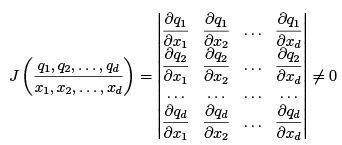The new qis need not even have the dimension of length (e.g. in spherical polar, the coordinates θ and φ are dimensionless.) This new set q1, q2,..........,qare known as the generalized coordinates.

Since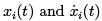are independentt of one another, the velocities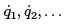are also independent set. These are known as the generalized velocities. [Lest there is confusion, I wish to emphasize that the number of generalized coordinates for a system with 3N degrees of freedom is 3N , it is only when the constraints are taken care of, it reduces to 3N k and one only needs these many independent coordinates to completely specify the system.]

We denote by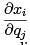the partial derivative of xi with respect to qj , when the remaining d 1 generalized coordinates are held xed.The real velocities associated with x= dxi/dt and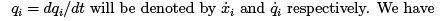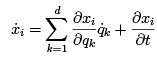If xi has no explicit time dependence (time dependence could arise, for instance, due to moving constraints), the last term of the above vanishes and we are left with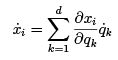which gives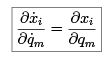This relationship is referred  as Dot Cancellation Theorem.
We will now show that the validity of the Euler Lagrange equation is not restricted to the Cartesian coordinates alone; they are equally valid in generalized coordinates. We have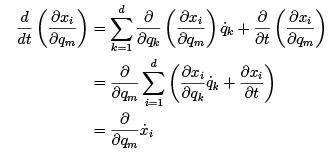(10)

We also have,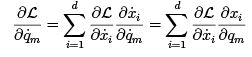(11)

where, in the last step, we have used in dot-cancellation relation. Taking time derivative of both sides of (11), we get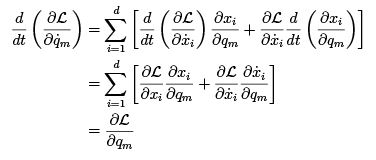(12)

since the last term is zero because the velocities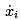are independent of the generalized coordinates. This establishes Euler Lagrange equation for the generalized coordinates.

The Brachistoschrone Problem

The word "Brachistochrone" is derived from the greek words brachistos meaning the shortest and chromous meaning time - i.e. it is about the shortest time problem.
Consider a bead sliding frictionlessly along a wire in a vertical plane from the point (x1, y1) to the point (x2, y2), where ylies lower than than y1. The problem is to design a path for which the time to slide is the shortest. Let us take the origin to be at the rst point (x1, y1) and take y to be positive downwards. The shape of the wire is given by y = f (x) ≥ 0. The wire is taken to lie below and to the right of the origin so that x ≥ 0 and y ≥ 0 along its path.
The time to come down is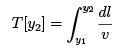The speed of the bead as it comes down is determined by the conservation of energy. Since y is positive downwards, the total energy must remain zero if the potential energy is taken to be zero at the origin. Thus we must have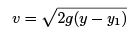Thus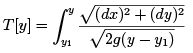It is convenient to convert this to an integral over y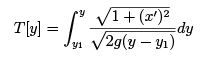where x' = dx/dy. Thus the Lagrangian function is a function of x' and y.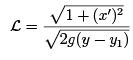Euler-Lagrange equation is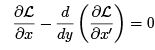As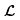has no dependence on x, the rst term of the above vanishes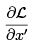is constant.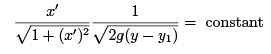so that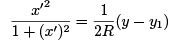where we have, for reasons to become clear later, chosen the constant to be 1=2R.
This gives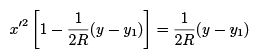resulting in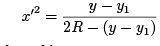We can parameterise the above by taking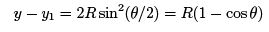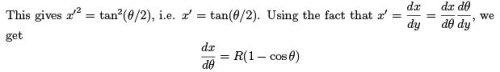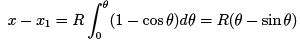Thus the shortest path is given by the pair of parameterised equations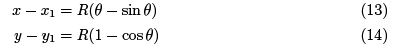These are parametric equations for a cycloid.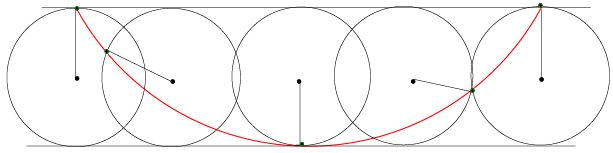Fig. 5: The path of a brachistochrone is shown in red

The lowest point of the brachistochrone corresponds to θ = π. The path is a curve traced out by a xed point on the circumference of a circle of radius R as the circular disk moves on a horizontal
oor. The constant R is determined by the condition that the curve must pass through the point y = y2. Thus if y2 is the lowest point then y2 y1 = 2R. an interesting point is that the time taken to descend to the lowest point is independent of the point from which it was released, i.e., it speeds up appropriately to cover the distance to the lowest position in the same time. The time is calculated as follows: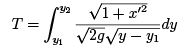With the above substitution, i.e.,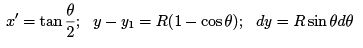we have,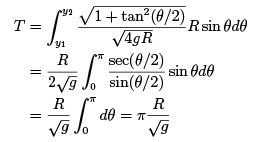(15)

The Spring Pendulum

As a second example, we consider a spring pendulum, in which the bob of the pendulum is connected to the support not by means of an inextensible string but by means of a spring whose length can only vary along its length (this can be achieved by encasing the spring in tube, for instance).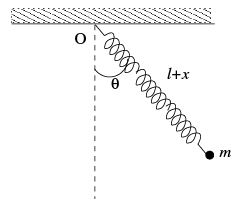Let the instantaneous distance of the bob from the support be l + x, where l is the natural length of the spring. We choose x to be one of the generalized coordinates and the angle θ that the spring makes with the vertical as the other (note that unlike the case of simple pendulum which only had one degree of freedom, we now have two). The kinetic
and the potential energies of the system are given in terms of these coordinates and the corresponding velocities as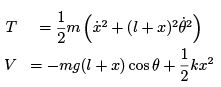The Lagrangian is of course, L = T − V . The Euler- Lagrange equations for the x coordinates are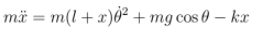The equation is readily identifiable as the equation for the radial acceleration with the first term on the right being the centripetal acceleration term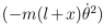which should be taken to the left to give the total radial acceleration. The second term is the radial component of the weight and the third term is the spring force. The equation (16) can be interpreted in the rotating frame in which case the first term gives the centrifugal force. The equation for the θ coordinate is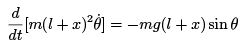(17)

The right hand side of this equation is the torque of the weight about the point of suspension. The left hand side is the rate of change of the angular momentum as can be easily seen, since the tangential velocity is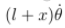and the distance from the support is (l + x). Explicitly differentiating (17) and cancelling a common factor, we can rewrite this equation as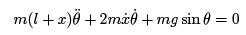This can be interpreted in the rotating frame, the rst term being the angular acceleration term and the second the Coriolis term.

159 docs

,

,

,

,

,

,

,

,

,

,

,

,

,

,

,

,

,

,

,

,

,

;